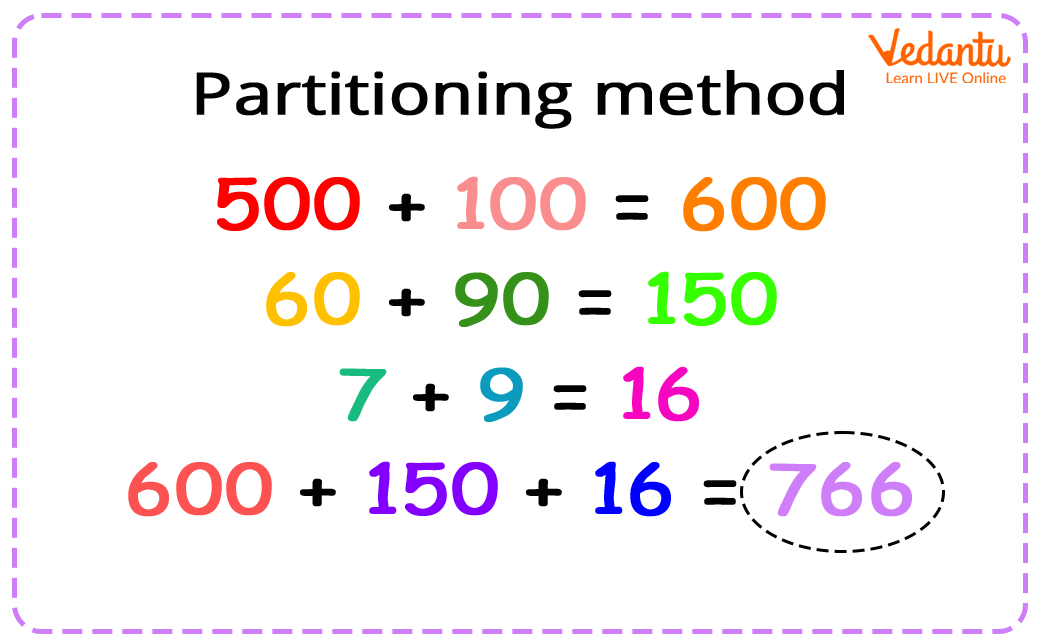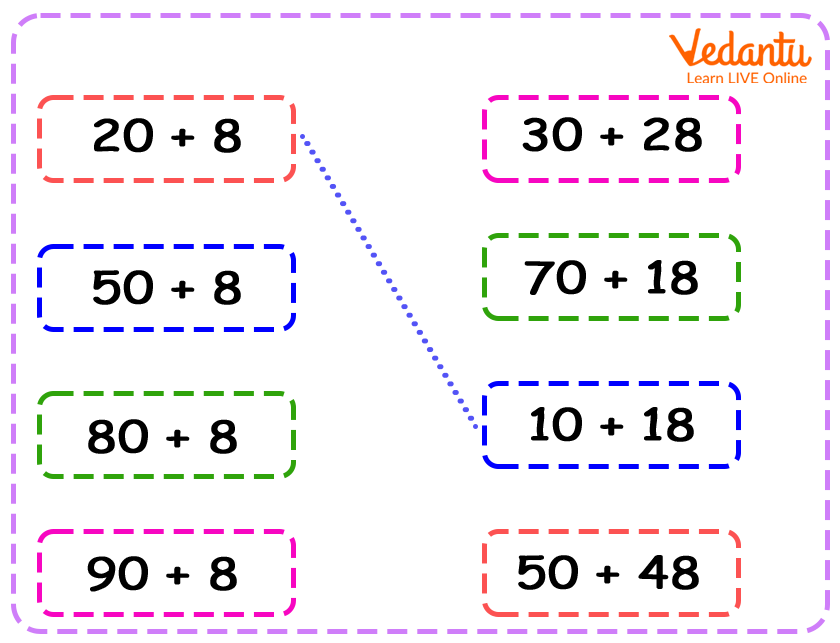Courses
Courses for Kids
Free study material
Free LIVE classes
More

# What Does Partition Mean in Maths?## Introduction

Partitioning is a concept that is generally picked up by kids at a very young age. We encourage parents to make their kids learn the idea behind partitioning so that they are able to work with numbers easily and specifically. So, when faced with the eventual question, what does partition mean in maths, the simple answer is that partitioning basically means splitting a number into smaller parts, which makes it easier to work with. Let us understand why partitioning is important in maths and look into some examples related to the concept.

## Importance of Partitioning in Maths

Partitioning plays a significant role in mathematics because it helps us break a problem or number into smaller parts that are easier to deal with. This is the simpler explanation of the idea. Now, if we are faced with a problem where the entire operation is too large to handle at one go, we resort to partitioning to dismantle the larger piece into smaller pieces to address them individually and then arrive at a final conclusion.

Children should be introduced to this concept of partitioning so that they are not afraid to deal with larger numbers or larger problems and can easily break them down as and when needed. It is also very important to understand that partitioning a number of problems is not always restricted to one single way, it can be done in multiple ways. It is the duty of parents and teachers to make children understand and be aware of these ways so that they can easily switch between the different approaches when need be.

A simple method of partitioning is to break down a number into numbers that can be added to arrive at the given number. The image below shows an appropriate application of such a method to break the numbers down.## Examples of Partitioning in Maths

Let us consider the following examples to better understand the ways in which a number can be partitioned:

1. Let us try to break the number 56 down into two parts each time.

• 56 = 50 + 6

• 56 = 40 + 16

• 56 = 30 + 26

• 56 = 20 + 36

• 56 = 10 + 46

• 56 = 6 + 50

There are many other ways to break the number 56 down into smaller parts.

1. Let us try to break the same number, 56, down into three parts every time.

• 56 = 40 + 10 + 6

• 56 = 30 + 20 + 6

• 56 = 20 + 30 + 6

• 56 = 10 + 40 + 6

• 56 = 6 + 10 + 40

In this way, 56 can be broken down into smaller numbers of 4, 5, 6 portions, and so on.## Conclusion

The concept of partitioning goes beyond the simple method of breaking a number down into addends. However, for kids to reap the benefits of partitioning in maths, the method given in this article should suffice and they should be able to solve problems in exams using the lesson learnt under this topic. Parents are advised to make their kids better internalise this concept by giving them real-world problems to solve, like asking them to divide a few fruits among their friends or siblings, and so on.

Last updated date: 26th Sep 2023
Total views: 138.9k
Views today: 4.38k

## FAQs on What Does Partition Mean in Maths?

1. What are some of the ways to partition the number 68?

The number 68 can be partitioned in some of the following ways:

• 68 = 60 + 8

• 68 = 60 + 4 + 4

• 68 = 30 + 30 + 8

• 68 = 20 + 20 + 20 + 8

2. What are the other ways of partitioning apart from breaking a number down into addends?

Some other ways of partitioning are breaking the number down into numbers involving operations like subtraction, multiplication, and division.